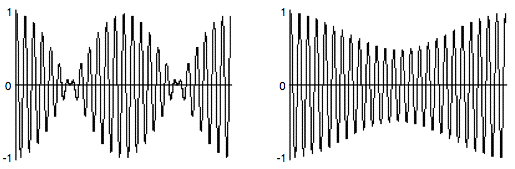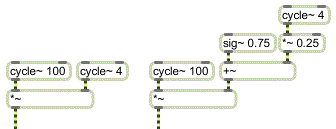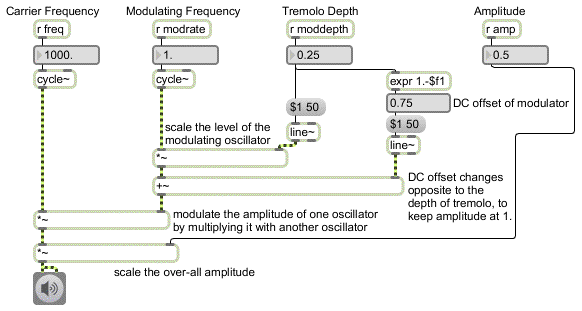Tutorial 9: Amplitude Modulation
Ring modulation and amplitude modulation
Amplitude modulation (AM) involves changing the amplitude of a ‘carrier’ signal using the output of another ‘modulator’ signal. In the specific AM case of ring modulation (discussed in Tutorial 8) the two signals are simply multiplied. In the more general case, the modulator is used to alter the carrier's amplitude, but is not the sole determinant of it. To put it another way, the modulator can cause fluctuation of amplitude around some value other than 0. The example below illustrates the difference between ring modulation and more common amplitude modulation.Ring modulation Amplitude modulation
The example on the left is 1/4 second of a 100 Hz cosine multiplied by a 4 Hz cosine; the amplitude of both cosines is 1. In the example on the right, the 4 Hz cosine has an amplitude of 0.25, which is used to vary the amplitude of the 100 Hz tone ±0.25 around 0.75 (going as low as 0.5 and as high as 1.0). The two main differences are a) the AM example never goes all the way to 0, whereas the ring modulation example does, and b) the ring modulation is perceived as two amplitude dips per modulation period (thus creating a tremolo effect at twice the rate of the modulation) whereas the AM is perceived as a single cosine fluctuation per modulation period.

The two MSP patches that made these examples are shown below.Ring modulation Amplitude modulation
The difference in effect is due to the constant value of 0.75 in the AM patch, which is varied by a modulator of lesser amplitude. This constant value can be thought of as the carrier's amplitude, which is varied by the instantaneous amplitude of the modulator. The amplitude still varies according to the shape of the modulator, but the modulator is not centered on 0.
Technical detail: The amount that a wave is offset from 0 is called the DC offset. A constant amplitude value such as this represents spectral energy at the frequency 0 Hz. The modulator in AM has a DC offset, which distinguishes it from ring modulation.

Implementing AM in MSP
The tutorial patch is designed in such a way that the DC offset of the modulator is always 1 minus the amplitude of its sinusoidal variation. That way, the peak amplitude of the modulator is always 1, so the product of carrier and modulator is always 1. A separate *~ object is used to control the over-all amplitude of the sound.The modulator is a sinusoid with a DC offset, which is multiplied by the carrier
• Click on the ezdac~ to turn audio on. Notice how the tremolo rate is the same as the frequency of the modulator. Click on the message boxes 2, 4, and 8 in turn to hear different tremolo rates.
Achieving different AM effects
The primary merit of AM lies in the fact that the intensity of its effect can be varied by changing the amplitude of the modulator.
• To hear a very slight tremolo effect, type the value 0.03 into the number box marked ‘Tremolo Depth’. The modulator now varies around 0.97, from 1 to 0.94, producing an amplitude variation of only about half a decibel. To hear an extreme tremolo effect, change the tremolo depth to 0.5; the modulator now varies from 1 to 0 -- the maximum modulation possible.
Amplitude modulation produces sidebands -- additional frequencies not present in the carrier or the modulator -- equal to the sum and the difference of the frequencies present in the carrier and modulator. The presence of a DC offset (technically energy at 0 Hz) in the modulator means that the carrier tone remains present in the output, too (which is not the case with ring modulation).
• Click on the message boxes containing the numbers 32, 50, 100, and 150, in turn. You will hear the carrier frequency, the modulator frequency (which is now in the low end of the audio range), and the sum and difference frequencies.
When there is a harmonic relationship between the carrier and the modulator, the frequencies produced belong to the harmonic series of a common fundamental, and tend to fuse more as a single complex tone. For example, with a carrier frequency of 1000 Hz and a modulator at 250 Hz, you will hear the frequencies 250 Hz, 750 Hz, 1000 Hz, and 1250 Hz— the 1st, 3rd, 4th, and 5th harmonics of the fundamental at 250 Hz.
• Click on the message boxes containing the numbers 200, 250, and 500 in turn to hear harmonic complex tones. Drag on the ‘Tremolo Depth’ number box to change the depth value between 0. and 0.5, and listen to the effect on the relative strength of the sidebands.
• Explore different possibilities by changing the values in the number box objects. When you have finished, click on the ezdac~ to turn audio off.
It is worth noting that any audio signals can be used as the carrier and modulator tones, and in fact many interesting results can be obtained by amplitude modulation with complex tones. (Tutorial 23 allows you to perform amplitude modulation on the sound coming into the computer.)
Summary
The amplitude of an audio (carrier) signal can be modulated by another (modulator) signal, either by simple multiplication (ring modulation) or by adding a time-varying modulating signal to a constant signal (DC offset) before multiplying it with the carrier signal (amplitude modulation). The intensity of the amplitude modulation can be controlled by increasing or reducing the amplitude of the time-varying modulator relative to its DC offset. When the modulator has a DC offset, the carrier frequency will remain present in the output sound, along with sidebands at frequencies determined by the sum and the difference of the carrier and the modulator. At sub-audio modulating frequencies, amplitude modulation is heard as tremolo; at audio frequencies the carrier, modulator, and sidebands are all heard as a chord or as a complex tone.